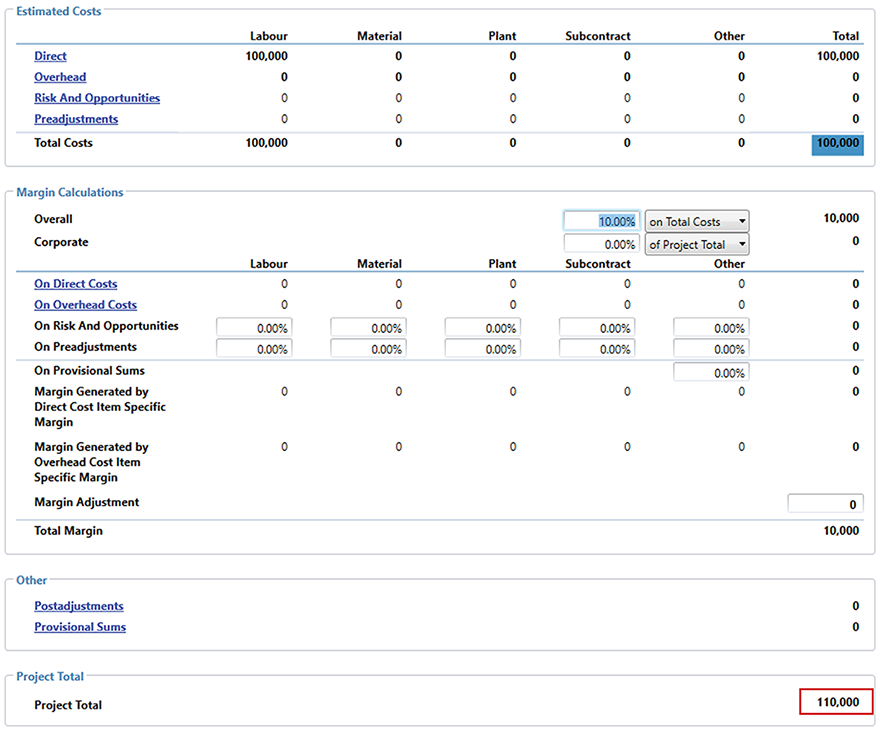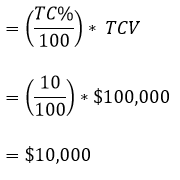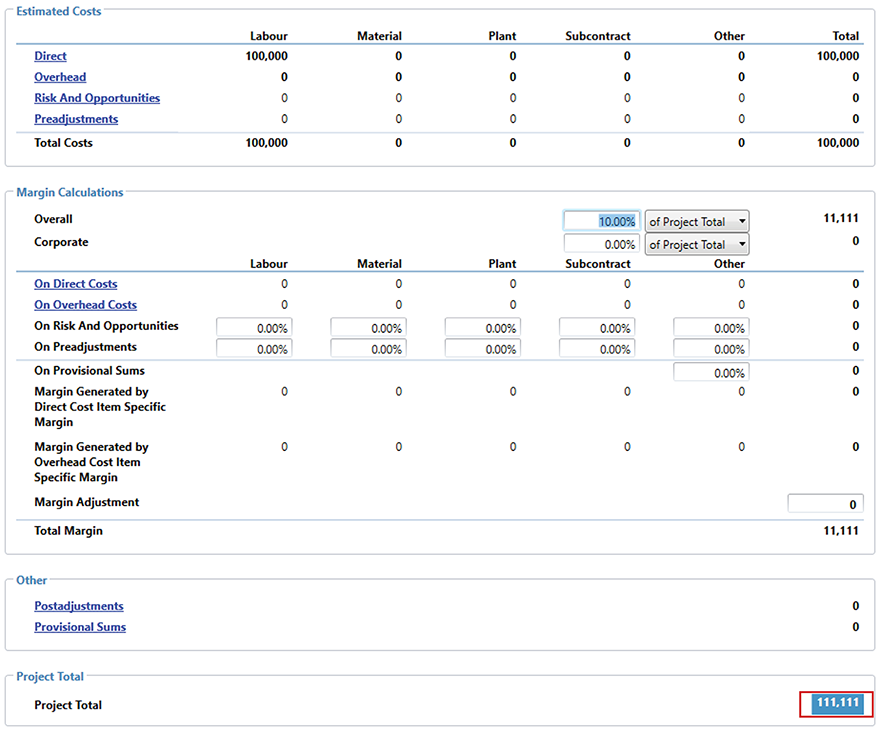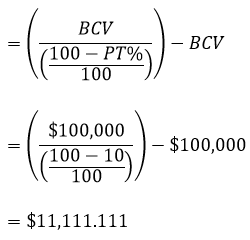## Project Summary – Margin Calculations

Expert Estimation defines two individual margin types that can be applied to a Project. These margin types, known as Overall and Corporate Margin, are both applied as percentages from within the Project Summary window.

Overall Margin allows you to record your profit margin for the project and is the most common way to generate margin for a project. Corporate Margin is used in conjunction with the Overall Margin and can represent a company standard margin that needs to be applied.

Note: Corporate Margin is available in Genesis and Express editions only.

Both of these margins can be configured to generate a total contributing margin based on either the Total Estimated Costs of the project or the final Project Total.

• Selecting on Total Costs tells Expert Estimation to mark up the Total Cost value by the percent specified. This is the default option set for both Overall and Corporate margins.
• Selecting of Project Total will generate the Overall margin by the specified percentage of the final Project Total.

The Overall and Corporate margin calculations are done independently of each other rather than being combined and then calculated, however, when calculating margin of Project Total you can choose to have the calculations combined. This setting is definable in the Project Properties – Calculations window.

#### ON TOTAL COST

The on Total Cost markup generates margin based on a percentage of the Total Costs. This is the default margin type and is calculated by entering a percentage, which is used to calculate a factored amount of the project’s Total Costs.

The image below shows the Total Cost of the project as \$100,000 (highlighted in dark blue) and the markup percentage is 10% on Total Cost (highlighted in light blue). The resulting margin value is \$10,000. This value acts as the Total Margin for the Project. It is this Total Margin plus the Total Costs that are then added together to achieve the final Project Total of \$110,000.Calculating margin based on Total Cost

The formula below shows how the total value of margin is calculated when using the margin type on Total Cost:

• TC% = The percentage specified on Total Cost = 10%
• TCV = Total Cost Value = Direct Total + Overhead Total + Risk and Opportunities Total + Preadjustments Total = \$100,000#### OF PROJECT TOTAL

The percentage of Project Total markup differs from on Total Cost by calculating… the value of margin where it represents a percentage of the overall contract, rather than a percentage on top of the total cost of the project.

Essentially, if the On Contract Value percentage is set to 10%, then that line of margin represents 10% of the contract.Calculating margin of Project Total

The formula below shows how the total value of margin is calculated when using the margin type of Project Total:

• PT% = The percentage specified of Project Total = 10%
• BCV = Base Contract Value = Total Cost Value + Direct Costs Margin + Overhead Costs Margin + Risk and Opportunities Margin + Preadjustments Margin + Provisional Sums Margin + Post margin Adjustment + Specific Margins + Margin Adjustment + Provisional Sums Total (if Calculation option has been set) = \$100,000